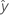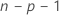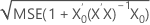# Methods and formulas for the fits and residuals in Analyze Response Surface Design

Select the method or formula of your choice.

## Fit

### Notation

TermDescriptionfitted value
xkkth term. Each term can be a single predictor, a polynomial term, or an interaction term.
bkestimate of kth regression coefficient

## Standard error of fitted value (SE Fit)

The standard error of the fitted value in a regression model with one predictor is:

The standard error of the fitted value in a regression model with more than one predictor is:

For weighted regression, include the weight matrix in the equation:

When the data have a test data set or K-fold cross validation, the formulas are the same. The value of s2 is from the training data. The design matrix and the weight matrix are also from the training data.

### Notation

TermDescription
s2mean square error
nnumber of observations
x0new value of the predictormean of the predictor
xiith predictor value
x0 vector of values that produce the fitted values, one for each column in the design matrix, beginning with a 1 for the constant term
x'0transpose of the new vector of predictor values
Xdesign matrix
Wweight matrix

## Residuals

The residual is the difference between an observed value and the corresponding fitted value. This part of the observation is not explained by the model. The residual of an observation is:

### Notation

TermDescription
yiith observed response valueith fitted value for the response

## Standardized residual (Std Resid)

Standardized residuals are also called "internally Studentized residuals."

### Notation

TermDescription
ei i th residual
hi i th diagonal element of X(X'X)–1X'
s2 mean square error
Xdesign matrix
X'transpose of the design matrix

## Deleted (Studentized) residuals

Also called the externally Studentized residuals. The formula is:

Another presentation of this formula is:

The model that estimates the ith observation omits the ith observation from the data set. Therefore, the ith observation cannot influence the estimate. Each deleted residual has a student's t-distribution withdegrees of freedom.

### Notation

TermDescription
eiith residual
s(i)2mean square error calculated without the ith observation
hi i th diagonal element of X(X'X)–1X'
nnumber of observations
pnumber of terms, including the constant
SSEsum of squares for error

## Confidence interval

The range in which the estimated mean response for a given set of predictor values is expected to fall. The interval is defined by lower and upper limits, which Minitab calculates from the confidence level and the standard error of the fits.

where

### Notation

TermDescription
αchosen alpha value
nnumber of observations
pnumber of parameters
s2mean square error
s2{b}variance-covariance matrix of the coefficients

## Prediction interval

The range in which the predicted response for a new observation is expected to fall. The interval is defined by lower and upper limits, which Minitab calculates from the confidence level and the standard error of the prediction. The prediction interval is always wider than the confidence interval because of the added uncertainty involved in predicting a single response versus the mean response.

The formula is:0+ t(1 -α /2; n - p) s(pred)

### Notation

TermDescription
αchosen alpha value
nnumber of observations
pnumber of predictors
s (prediBy using this site you agree to the use of cookies for analytics and personalized content.  Read our policy